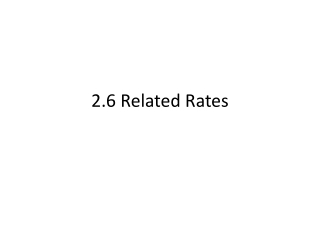DownloadDownload Presentation2.6 Related Rates

# 2.6 Related Rates

Télécharger la présentation## 2.6 Related Rates

- - - - - - - - - - - - - - - - - - - - - - - - - - - E N D - - - - - - - - - - - - - - - - - - - - - - - - - - -
##### Presentation Transcript

1. 2.6 Related Rates

2. Two Rates that are Related • Suppose x and y are both differentiable functions of t and are related by the equation given. Find dy/dt when x=1, given that dx/dt=2 when x=1.

3. Ripples in a pond • A pebble is dropped into a calm pond, causing ripples in the form of concentric circles. The radius of the outer ripple is increasing at a constant rate of 1 foot per second. When the radius is 4 feet, at what rate is the area A of the disturbed water changing?

4. An Inflating Balloon • Air is being pumped into a spherical balloon at a rate of 4.5 cubic feet per minute. Find the rate of change of the radius when the radius is 2 feet.

5. The Speed of an Airplane Tracked by Radar • An airplane is flying on a flight path that will take it directly over a radar tracking station. If s is decreasing at a rate of 400 miles per hour when s=10 miles, what is the speed of the plane? s 6 mi x

6. A Changing Angle of Elevation • Find the rate of change in the angle of elevation of the camera shown in the picture at 10 seconds after lift off. s θ 2000 ft